# 基于激光非线性的谐波通项推导和nf/1f免标应用Deduction of General Term for Harmonics and Application of Calibration-Free nf/1f Models Based on the Lazer Nonlinearity

• 全文下载: PDF(2714KB)    PP.91-102   DOI: 10.12677/APP.2020.101011
• 下载量: 111  浏览量: 1,126   国家自然科学基金支持

The nonlinearity of the laser frequency and intensity response in the wavelength modulation spectroscopy (WMS) was analyzed through the high-accuracy experiments. By full consideration of the nonlinear effect, the nf harmonic, and thus the calibration-free nf/1f model were further deduced based on the absorption spectrum and harmonic theory. Moreover, a practical and sim-plified nf/1f calibration-free method was established based on the second-order approximation of the laser frequency and intensity. As a verification of the proposed method, the CO transition at 4300.699 cm−1 was investigated under both strong and weak absorption conditions. Meanwhile, the CO concentrations were measured with the proposed method and compared with those ignoring the nonlinear effect. The measurement result shows that the accuracy of the gas concentration measurement can be effectively improved with consideration of the nonlinearity of laser intensity and frequency. In addition, the CO concentration can be measured with high precision (relative error < 0.5%) by using the quadratic approximation 2f/1f, 4f/1f and 6f/1f methods.

1. 引言

2. 激光频率和光强非线性效应分析

$\left\{\begin{array}{l}\upsilon \left(t\right)=\stackrel{¯}{\upsilon }+\underset{j=1}{\overset{{n}_{\upsilon }}{\sum }}{a}_{j}\mathrm{cos}\left(j{\omega }_{m}t+{\phi }_{j}\right)\\ {I}_{0}=\stackrel{¯}{I}\left[1+\underset{j=1}{\overset{{n}_{I}}{\sum }}{i}_{j}\mathrm{cos}\left(j{\omega }_{m}t+{\psi }_{j}\right)\right]\end{array}$ (1)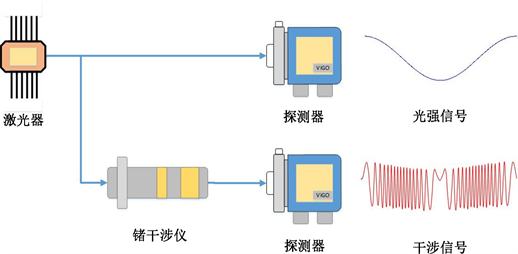Figure 1. Calibration experiment of laser frequency and intensity nonlinearty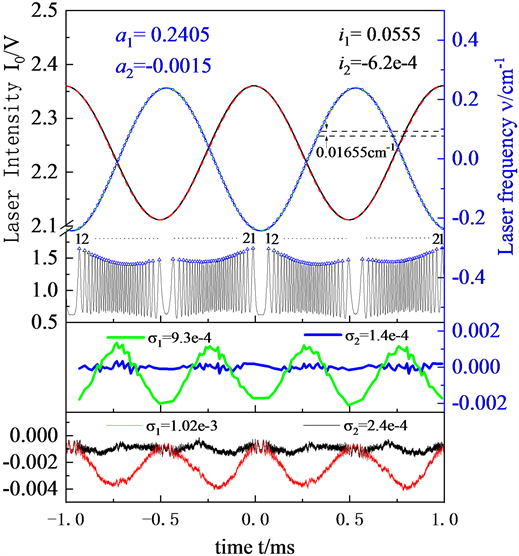Figure 2. Calibration results of laser frequency and intensity nonlineart

3. 基于激光非线性效应的谐波理论

3.1. 通项表达式推导

$\tau \left(\nu \left(t\right)\right)=\frac{{I}_{t}}{{I}_{0}}=\mathrm{exp}\left[-A\phi \left(\nu \left(t\right)\right)\right]=\mathrm{exp}\left[-LS\left(T\right)XP\phi \left(\nu \left(t\right)\right)\right]$ (2)

$\tau \left(t\right)=\underset{k=0}{\overset{\infty }{\sum }}{H}_{k}\mathrm{cos}\left(k{\omega }_{m}t\right)+{J}_{k}\mathrm{cos}\left(k{\omega }_{m}t\right)$ (3)

$\left\{\begin{array}{l}{H}_{0}=\frac{1}{2\pi }{\int }_{-\pi }^{\pi }\tau \left(t\right)\text{d}t\text{\hspace{0.17em}}\text{\hspace{0.17em}}\text{\hspace{0.17em}}\text{\hspace{0.17em}}\text{\hspace{0.17em}}\text{\hspace{0.17em}}\text{\hspace{0.17em}}\text{\hspace{0.17em}}\text{\hspace{0.17em}}\text{\hspace{0.17em}}\text{\hspace{0.17em}}\text{\hspace{0.17em}}\text{\hspace{0.17em}}\text{\hspace{0.17em}}\text{\hspace{0.17em}}\text{\hspace{0.17em}}\text{\hspace{0.17em}}\text{\hspace{0.17em}}\text{\hspace{0.17em}}\text{\hspace{0.17em}}\text{\hspace{0.17em}}{J}_{0}=0\\ {H}_{k}=\frac{1}{\pi }{\int }_{-\pi }^{\pi }\tau \left(t\right)\mathrm{cos}\left(k{\omega }_{m}t\right)\text{d}t\text{\hspace{0.17em}}\text{\hspace{0.17em}}\text{\hspace{0.17em}}\text{\hspace{0.17em}}\text{\hspace{0.17em}}\text{\hspace{0.17em}}\text{\hspace{0.17em}}\text{\hspace{0.17em}}{J}_{k}=\frac{1}{\pi }{\int }_{-\pi }^{\pi }\tau \left(t\right)\mathrm{sin}\left(k{\omega }_{m}t\right)\text{d}t\end{array}$ (4)

${I}_{t}=\stackrel{¯}{{I}_{0}}\left[1+\underset{j=1}{\overset{{n}_{I}}{\sum }}{i}_{j}\mathrm{cos}\left(j{\omega }_{m}{\psi }_{j}\right)\right]\cdot \left[\underset{k=0}{\overset{\infty }{\sum }}{H}_{k}\mathrm{cos}\left(k{\omega }_{m}t\right)+{J}_{k}\mathrm{sin}\left(k{\omega }_{m}t\right)\right]$ (5)

$\left\{\begin{array}{l}{X}_{nf}=\frac{\pi }{{\omega }_{n}}{\int }_{-\frac{{\omega }_{n}}{\pi }}^{\frac{{\omega }_{n}}{\pi }}{I}_{t}\cdot \mathrm{cos}\left(n{\omega }_{m}t\right)\text{d}t\\ \text{\hspace{0.17em}}\text{\hspace{0.17em}}\text{\hspace{0.17em}}\text{\hspace{0.17em}}\text{\hspace{0.17em}}\text{\hspace{0.17em}}=\stackrel{¯}{{I}_{0}}\left[{H}_{n}+{H}_{-n}+\underset{j=1}{\overset{{n}_{I}}{\sum }}\frac{{i}_{j}}{2}\left(\left({H}_{j+n}+{H}_{j-n}+{H}_{-j+n}+{H}_{-j-n}\right)\mathrm{cos}{\psi }_{j}\\ \text{\hspace{0.17em}}\text{\hspace{0.17em}}\text{\hspace{0.17em}}\text{\hspace{0.17em}}\text{\hspace{0.17em}}\text{\hspace{0.17em}}\text{\hspace{0.17em}}\begin{array}{c}\text{ }\\ \text{ }\end{array}+\left(-{J}_{j+n}-{J}_{j-n}+{J}_{-j+n}+{J}_{-j-n}\right)\mathrm{sin}{\psi }_{j}\right)\right]\\ {Y}_{nf}=\frac{\pi }{{\omega }_{n}}{\int }_{-\frac{{\omega }_{n}}{\pi }}^{\frac{{\omega }_{n}}{\pi }}{I}_{t}\cdot \mathrm{sin}\left(n{\omega }_{m}t\right)\text{d}t\\ \text{\hspace{0.17em}}\text{\hspace{0.17em}}\text{\hspace{0.17em}}\text{\hspace{0.17em}}\text{\hspace{0.17em}}=\stackrel{¯}{{I}_{0}}\left[{J}_{n}+\underset{j=1}{\overset{{n}_{I}}{\sum }}\frac{{i}_{j}}{2}\left[\left(-{H}_{j+n}+{H}_{j-n}+{H}_{-j+n}-{H}_{-j-n}\right)\mathrm{sin}{\psi }_{j}\\ \text{\hspace{0.17em}}\text{\hspace{0.17em}}\text{\hspace{0.17em}}\text{\hspace{0.17em}}\text{\hspace{0.17em}}\text{\hspace{0.17em}}\begin{array}{c}\text{ }\\ \text{ }\end{array}+\left({J}_{j+n}-{J}_{j-n}+{J}_{-j+n}-{J}_{-j-n}\right)\mathrm{cos}{\psi }_{j}\right]\right]\\ {R}_{nf}=\sqrt{{X}_{nf}^{2}+{Y}_{nf}^{2}}\end{array}$ (6)

3.2. 基于二阶近似的nf/1f免标模型

$\left\{\begin{array}{l}\upsilon \left(t\right)=\stackrel{¯}{\upsilon }+{a}_{1}\mathrm{cos}\left({\omega }_{m}t+{\phi }_{1}\right)+{a}_{2}\mathrm{cos}\left(\text{2}{\omega }_{m}t+{\phi }_{2}\right)\\ {I}_{0}=\stackrel{¯}{{I}_{0}}\left[1+{i}_{1}\mathrm{cos}\left({\omega }_{m}t+{\psi }_{1}\right)+{i}_{2}\mathrm{cos}\left(\text{2}{\omega }_{m}t+{\psi }_{2}\right)\right]\end{array}$ (7)

$\left\{\begin{array}{l}{X}_{nf}=\stackrel{¯}{{I}_{0}}\left[{H}_{n}+\frac{{i}_{1}}{2}\left(\left({H}_{n+1}+{H}_{1-n}+{H}_{n-1}\right)\mathrm{cos}{\psi }_{1}+\left(-{J}_{n+1}-{J}_{1-n}+{J}_{n-1}\right)\mathrm{sin}{\psi }_{1}\right)\\ \text{\hspace{0.17em}}\text{\hspace{0.17em}}\text{\hspace{0.17em}}\text{\hspace{0.17em}}\text{\hspace{0.17em}}\text{\hspace{0.17em}}\text{\hspace{0.17em}}\text{\hspace{0.17em}}+\frac{{i}_{2}}{2}\left(\left({H}_{n+2}+{H}_{2-n}+{H}_{n-2}\right)\mathrm{cos}{\psi }_{2}+\left(-{J}_{n+2}-{J}_{2-n}+{J}_{n-2}\right)\mathrm{sin}{\psi }_{2}\right)\right]\\ {Y}_{nf}=\stackrel{¯}{{I}_{0}}\left[{J}_{n}+\frac{{i}_{1}}{2}\left(\left(-{H}_{n+1}+{H}_{1-n}+{H}_{n-1}\right)\mathrm{sin}{\psi }_{1}+\left({J}_{n+1}-{J}_{1-n}+{J}_{n-1}\right)\mathrm{cos}{\psi }_{1}\right)\\ \text{\hspace{0.17em}}\text{\hspace{0.17em}}\text{\hspace{0.17em}}\text{\hspace{0.17em}}\text{\hspace{0.17em}}\text{\hspace{0.17em}}\text{\hspace{0.17em}}\text{\hspace{0.17em}}+\frac{{i}_{2}}{2}\left(\left(-{H}_{n+2}+{H}_{2-n}+{H}_{n-2}\right)\mathrm{sin}{\psi }_{2}+\left({J}_{n+2}-{J}_{2-n}+{J}_{n-2}\right)\mathrm{cos}{\psi }_{2}\right)\right]\\ {R}_{nf}=\sqrt{{X}_{nf}^{2}+{Y}_{nf}^{2}}\end{array}$ (8)

$\left\{\begin{array}{l}{X}_{2f}=\stackrel{¯}{{I}_{0}}\left[{H}_{2}+\frac{{i}_{1}}{2}\left(\left({H}_{1}+{H}_{3}\right)\mathrm{cos}{\psi }_{1}+\left({J}_{1}-{J}_{3}\right)\mathrm{sin}{\psi }_{1}\right)\\ \text{\hspace{0.17em}}\text{\hspace{0.17em}}\text{\hspace{0.17em}}\text{\hspace{0.17em}}\text{\hspace{0.17em}}\text{\hspace{0.17em}}\text{\hspace{0.17em}}\text{\hspace{0.17em}}\text{\hspace{0.17em}}+\frac{{i}_{2}}{2}\left(\left(2{H}_{0}+{H}_{4}\right)\mathrm{cos}{\psi }_{2}-{J}_{4}\mathrm{sin}{\psi }_{2}\right)\right]\\ {Y}_{2f}=\stackrel{¯}{{I}_{0}}\left[{J}_{2}+\frac{{i}_{1}}{2}\left(\left({H}_{1}-{H}_{3}\right)\mathrm{sin}{\psi }_{1}+\left({J}_{1}+{J}_{3}\right)\mathrm{cos}{\psi }_{1}\right)\\ \text{\hspace{0.17em}}\text{\hspace{0.17em}}\text{\hspace{0.17em}}\text{\hspace{0.17em}}\text{\hspace{0.17em}}\text{\hspace{0.17em}}\text{\hspace{0.17em}}\text{\hspace{0.17em}}+\frac{{i}_{2}}{2}\left(\left(2{H}_{0}-{H}_{4}\right)\mathrm{sin}{\psi }_{2}+{J}_{4}\mathrm{cos}{\psi }_{2}\right)\right]\end{array}$ (9)

$\left\{\begin{array}{l}{X}_{\text{4}f}=\stackrel{¯}{{I}_{0}}\left[{H}_{4}+\frac{{i}_{1}}{2}\left(\left({H}_{3}+{H}_{5}\right)\mathrm{cos}{\psi }_{1}+\left({J}_{3}-{J}_{5}\right)\mathrm{sin}{\psi }_{1}\right)\\ \text{\hspace{0.17em}}\text{\hspace{0.17em}}\text{\hspace{0.17em}}\text{\hspace{0.17em}}\text{\hspace{0.17em}}\text{\hspace{0.17em}}\text{\hspace{0.17em}}\text{\hspace{0.17em}}\text{\hspace{0.17em}}+\frac{{i}_{2}}{2}\left(\left({H}_{2}+{H}_{6}\right)\mathrm{cos}{\psi }_{2}+\left({J}_{2}-{J}_{6}\right)\mathrm{sin}{\psi }_{2}\right)\right]\\ {Y}_{\text{4}f}=\stackrel{¯}{{I}_{0}}\left[{J}_{4}+\frac{{i}_{1}}{2}\left(\left({H}_{3}-{H}_{5}\right)\mathrm{sin}{\psi }_{1}+\left({J}_{3}+{J}_{5}\right)\mathrm{cos}{\psi }_{1}\right)\\ \text{\hspace{0.17em}}\text{\hspace{0.17em}}\text{\hspace{0.17em}}\text{\hspace{0.17em}}\text{\hspace{0.17em}}\text{\hspace{0.17em}}\text{\hspace{0.17em}}\text{\hspace{0.17em}}+\frac{{i}_{2}}{2}\left(\left({H}_{2}-{H}_{6}\right)\mathrm{sin}{\psi }_{2}+\left({J}_{2}+{J}_{6}\right)\mathrm{cos}{\psi }_{2}\right)\right]\end{array}$ (10)

4. 实验验证

4.1. 谱线选择和实验方案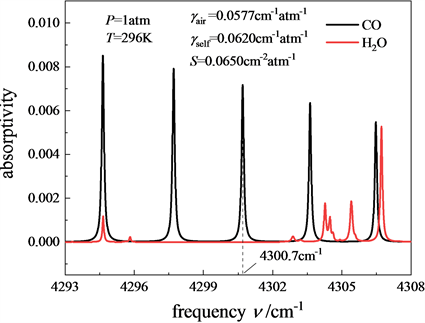Figure 3. Absorption lines of CO and H2O molecules near 4300 cm−1

4.2. 测量策略

$\left\{\begin{array}{l}\nu =\stackrel{¯}{\nu }+a\mathrm{cos}\left({\omega }_{0}t+\phi \right)\\ m=\frac{a}{\Delta \nu }\end{array}$ (10)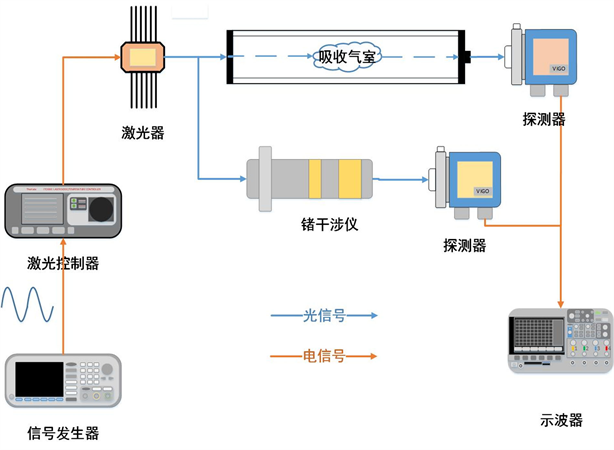Figure 4. Calibration-free nf/1f experiment based on nonlinearty of laser parameter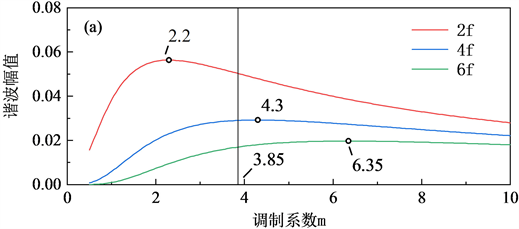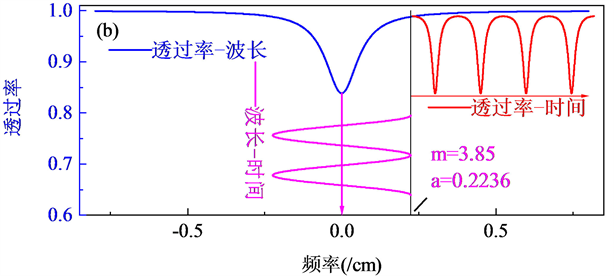Figure 5. (a) Relationship between the harmonic amplitude and modulation factor in Fix-WMS; (b) Principle of harmonic measurement in Fix-WMS

4.3. 实验结果分析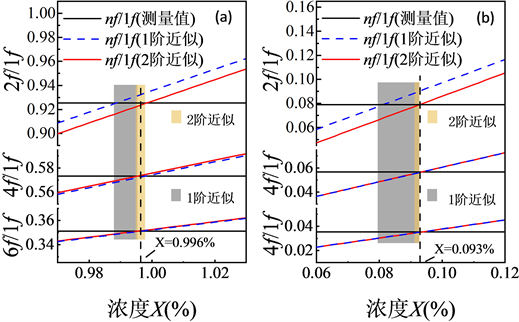Figure 6. Measurement results of calibration-free nf/1f (n = 2, 4, 6) based on second-order approximation (a) strong absorption (b) weak absorptionTable 1. Fitting concentration in different models and harmonics

5. 总结

  Werle, P.A. (1998) Review of Recent Advances in Semiconductor Laser Based Gas Monitors. Spectrochimica Acta Part A, 54, 197-236. https://doi.org/10.1016/S1386-1425(97)00227-8  Sur, R., Sun, K., Jeffries, J.B., Socha, J.G. and Hanson, R.K. (2015) Scanned-Wavelength-Modulation Spectroscopy Sensor for CO, CO2, CH4 and H2O in a High-Pressure Engineering-Scale Transport-Reactor Coal Gasifier. Fuel, 150, 102-111. https://doi.org/10.1016/j.fuel.2015.02.003  阚瑞峰, 刘文清, 张玉钧, 刘建国, 董凤忠, 高山虎, 王敏, 陈军. 可调谐二极管激光吸收光谱法测量环境空气中的甲烷含量[J]. 物理学报, 2005, 54(4): 1927-1930.  蓝丽娟, 丁艳军, 贾军伟, 杜艳君, 彭志敏. 可调谐二极管激光吸收光谱测量真空环境下气体温度的理论与实验研究[J]. 物理学报, 2014, 63(8): 083301-1-083301-9.  Peng, Z.M., Ding, Y.J. and Li, Z. (2013) First Harmonic with Wavelength Modulation Spectroscopy to Measure Integrated Absorbance under Low Absorption. Optics Express, 21, 23724-23725. https://doi.org/10.1364/OE.21.023724  Du, Y.J., Peng, Z.M. and Ding, Y.J. (2018) High-Accuracy Sinewave-Scanned Direct Absorption Spectroscopy. Optics Express, 26, 29550-29560. https://doi.org/10.1364/OE.26.029550  Reid, J. and Labrie, D. (1981) Second-Harmonic Detection with Tunable Diode-Lasers—Comparison of Experiment and Theory. Applied Physics B, 26, 203-210. https://doi.org/10.1007/BF00692448  蔡廷栋, 高光珍, 王敏锐, 王贵师, 高晓明. 高温高压下基于TD LAS的二氧化碳浓度测量方法研究[J]. 光谱学与光谱分析, 2014, 34(7): 1769-1773.  李劲松, 高晓明, 吴涛, 谈图, 方黎, 张为俊. 灵敏的基于分布反馈式半导体激光波长调制光声光谱[J]. 原子与分子物理学报, 2007, 24(1): 168-172.  Zhu, X. and Cassidy, D.T. (1997) Modulation Spectroscopy with a Semiconductor Diode Laser by Injection-Current Modulation. Journal of the Optical Society of America B, 14, 1945-1950. https://doi.org/10.1364/JOSAB.14.001945  Silver, J.A. (1992) Frequency-Modulation Spectroscopy for Trace Species Detection: Theory and Comparison among Experimental Methods. Applied Optics, 31, 707-717. https://doi.org/10.1364/AO.31.000707  Rieker, G.B., Jeffffries, J.B. and Hanson, R.K. (2009) Calibration-Free Wavelength-Modulation Spectroscopy for Measurements of Gas Temperature and Concentration in Harsh Environments. Applied Optics, 48, 5546-5560. https://doi.org/10.1364/AO.48.005546  Farooq, A., Jeffffries, J.B. and Hanson, R.K. (2009) Sensitive Detection of Temperature behind Reflected Shock Waves Using Wavelength Modulation Spectroscopy of CO2 Near 2.7 μm. Applied Physics B, 96, 161-173. https://doi.org/10.1007/s00340-009-3446-7  Cai, T.D., Gao, G.Z., Chen, W.D., Liu, G. and Gao, X.M. (2011) Simultaneous Measurements of CO2 and CO Using a Single Distributed-Feedback (DFB) Diode Laser near 1.57 μm at Elevated Temperatures. Applied Spectroscopy, 65, 108-112. https://doi.org/10.1366/10-06074  Li, H.J., Rieker, G.B., Liu, X., Jeffries, J.B. and Hanson, R.K. (2006) Extension of Wavelength-Modulation Spectroscopy to Large Modulation Depth for Diode Laser Absorption Measurements in High-Pressure Gases. Applied Optics, 45, 1052-1061. https://doi.org/10.1364/AO.45.001052  Chao, X., Jeffries, J.B., Hanson, R.K. (2012) Wavelength-Modulation-Spectroscopy for Real-Time, in Situ NO Detection in Combustion Gases with a 5.2 µm Quantum-Cascade Laser. Applied Physics B, 106, 987-997. https://doi.org/10.1007/s00340-011-4839-y  Sun, K., Chao, X., Sur, R., Goldenstein, C.S., Jeffries, J.B. and Hanson, R.K. (2013) Analysis of Calibration-Free Wavelengthscanned Wavelength Modulation Spectroscopy for Practical Gas Sensing Using Tunable Diode Lasers. Measurement Science and Technology, 24, Article ID: 125203. https://doi.org/10.1088/0957-0233/24/12/125203  Du, Y.J., Peng, Z.M. and Ding, Y.J. (2018) Wavelength Modulation Spectroscopy for Recovering Absolute Absorbance. Optics Express, 26, 9263-9272. https://doi.org/10.1364/OE.26.009263  Posener, D.W. (1959) The Shape of Spectral Lines: Tables of the Voigt Profile. Australian Journal of Physics, 12, 184-196. https://doi.org/10.1071/PH590184  聂伟, 阚瑞峰, 许振宇, 姚路, 夏晖晖, 彭于权, 张步强, 何亚柏. 基于TDLAS技术的水汽低温吸收光谱参数测量[J]. 物理学报. 2017, 66(20): 102-108.  Li, J.D., Du, Y.J., Peng, Z.M. and Ding Y.J. (2019) Measurements of Spectroscopic Parameters of CO2 Transitions for Voigt, Rautian, Galatry and Speed-Dependent Voigt Profiles Near 1.43 μm Using the WM-DAS Method. Journal of Quantitative Spectroscopy and Radiative Transfer, 224, 197-205. https://doi.org/10.1016/j.jqsrt.2018.11.014  Sun, K., Khan, A., Miller, D.J., Rafferty, K., Schreiber, J., Puzio, C., Portenti, M., Silver, J. and Zondlo, M.A. (2010) Open-Path Atmospheric N2O, CO, and NH3 Measurements Using Quantum Cascade Laser Spectroscopy. AGU Fall Meeting Abstracts, San Francisco, CA, B13E 08.  Lan, L.J., Ding, Y.J., Peng, Z.M., Du, Y.J. and Liu, Y.F. (2014) Calibration-Free Wavelength Modulation for Gas Sensing in Tunable Diode Laser Absorption Spectroscopy. Applied Physics B, 117, 1211-1219. https://doi.org/10.1007/s00340-014-5945-4  Goldenstein, C.S., Almodóvar, C.A., Jeffries, J.B., Hanson, R.K. and Brophy, C.M. (2014) High-Bandwidth Scanned- Wavelength Modulation Spectroscopy Sensors for Temperature and H2O in a Rotating Detonation Engine. Measurement Science and Technology, 25, Article ID: 105104. https://doi.org/10.1088/0957-0233/25/10/105104